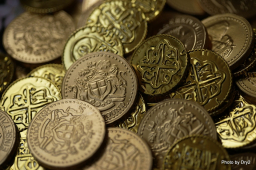# Dividing money 6

P 49,000 is to be divided among three children X, Y, and Z. If X will receive double that of Y. Y will receive double that of Z, how much will Z will receive?

Z =  7000

### Step-by-step explanation:Did you find an error or inaccuracy? Feel free to write us. Thank you!

Tips for related online calculators
Need help calculating sum, simplifying, or multiplying fractions? Try our fraction calculator.
Do you have a linear equation or system of equations and looking for its solution? Or do you have a quadratic equation?